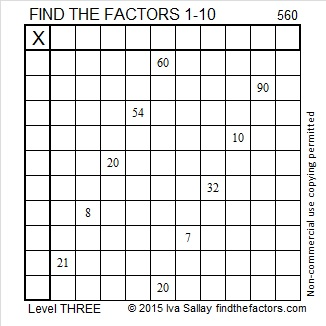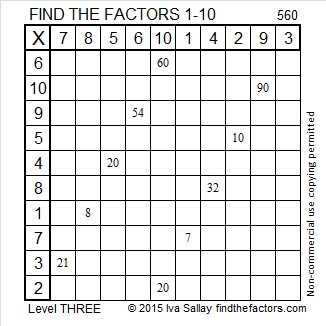# 560 and Level 3

560 is the hypotenuse of the Pythagorean triple 336-448-560. What is the greatest common factor of those three numbers?Print the puzzles or type the solution on this excel file: 10 Factors 2015-07-20

—————————————————————————————————

• 560 is a composite number.
• Prime factorization: 560 = 2 x 2 x 2 x 2 x 5 x 7, which can be written 560 = (2^4) x 5 x 7
• The exponents in the prime factorization are 4, 1 and 1. Adding one to each and multiplying we get (4 + 1)(1 + 1)(1 + 1) = 5 x 2 x 2 = 20. Therefore 560 has exactly 20 factors.
• Factors of 560: 1, 2, 4, 5, 7, 8, 10, 14, 16, 20, 28, 35, 40, 56, 70, 80, 112, 140, 280, 560
• Factor pairs: 560 = 1 x 560, 2 x 280, 4 x 140, 5 x 112, 7 x 80, 8 x 70, 10 x 56, 14 x 40, 16 x 35 or 20 x 28
• Taking the factor pair with the largest square number factor, we get √560 = (√16)(√35) = 4√35 ≈ 23.664319—————————————————————————————————

A Logical Approach to solve a FIND THE FACTORS puzzle: Find the column or row with two clues and find their common factor. Write the corresponding factors in the factor column (1st column) and factor row (top row).  Because this is a level three puzzle, you have now written a factor at the top of the factor column. Continue to work from the top of the factor column to the bottom, finding factors and filling in the factor column and the factor row one cell at a time as you go.